# Study of a particle swarm optimization method with multidirectional competition for the ground target attacking weapon-target allocation problem

London Journal of Engineering Research
Volume | Issue | Compilation
Authored by Shi , NA
Classification: For Code: 291899p
Keywords: weapon-target allocation; particle swarm optimization algorithm; multidirectional competition; affinity propagation clustering algorithm; genetic algorithm
Language: English

Key ground targets and ground target attacking weapon types are complex and diverse; thus, the weapon-target allocation (WTA) problem has long been a great challenge but has not yet been adequately addressed. A timely and reasonable WTA scheme not only helps to seize a fleeting combat opportunity but also optimizes the use of weaponry resources to achieve maximum battlefield benefits at the lowest cost. In this study, we constructed a ground target attacking WTA (GTA-WTA) model and proposed a particle swarm optimization method with multidirectional competition. The method uses an affinity propagation (AP) clustering algorithm to detect the location of the local optimal regions in the search space, introduces a multidirectional competition factor in the speed update of the particle swarm optimization (PSO) algorithm to guide the particles to compete and search in multiple directions to avoid the algorithm falling into a local optimum, thus accelerating the optimization. The simulation results show that the proposed method can greatly improve the solving efficiency of the GTA-WTA problem, and the obtained allocation scheme is also superior, and the larger the problem scale, the more obvious the effect.

# Study of a Particle Swarm Optimization Method with Multidirectional Competition for the Ground Target Attacking Weapon-Target Allocation Problem

## Guangyuan Fuα, Chao Wangσ, Daqiao Zhangρ, Ranhui WangѠ& Jiufen Zhao¥____________________________________________

### ABSTRACT

Key ground targets and ground target attacking weapon types are complex and diverse; Thus, the weapon-target allocation (WTA) problem has long been a great challenge but has not yet been adequately addressed. A timely and reasonable WTA scheme not only helps to seize a fleeting combat opportunity but also optimizes the use of weaponry resources to achieve maximum battlefield benefits at the lowest cost. In this study, we constructed a ground target attacking WTA (GTA-WTA) model and proposed a particle swarm optimization method with the multidirectional competition. It uses an affinity propagation (AP) clustering algorithm to detect the location of the local optimal regions in the search space, introduces a multidirectional competition factor in the speed update of the particle swarm optimization (PSO) algorithm to guide the particles to compete in multiple directions and to avoid the algorithm falling into a local optimum, thus accelerating the optimization. The simulation results show that the proposed method can significantly improve the solving efficiency of the GTA-WTA problem, and the obtained allocation scheme is also superior, and the larger the problem scale, the more obvious the effect.

Keywords: weapon-target allocation; particle swarm optimization algorithm; multidirectional competition; affinity propagation clustering algorithm; genetic algorithm.

Author α σ ρѠ ¥:  Xi’an Institute of High-Tech, Xi’an 710025, China.

1.  INTRODUCTION

WTA [1, 2] is a critical link in the operational command that directly affects the operational process and outcome and is thus a main military issue that has attracted the attention of military powers worldwide. Many achievements in the study of WTA, mostly regarding the air defense WTA (AD-WTA), which addresses air defense interception [3-14], whereas the GTA-WTA problem, which addresses ground targets, has rarely been studied [15-18]. Compared with air defense interception, ground targets are diverse and suitable for many weaponry types, so the scale of the WTA problem is wider and more complex, leading to the existing AD-WTA algorithm is inadequate to solve a GTA-WTA problem.

Currently, the optimization algorithms for a GTA-WTA problem primarily include intelligent algorithms such as the PSO algorithm [15, 16], the genetic algorithm (GA) , and the bat algorithm . To address the GTA-WTA problem, Wang et al.  improved the particle initialization and weight coefficient selection methods of the PSO algorithm, which improved the computational efficiency and the solution quality. Wang et al.  proposed an improved GA combining the adaptive GA with the variable domain searching algorithm, which can balance the global and local search abilities of the algorithm and achieve intended results. Liu et al.  proposed an improved bat algorithm that integrated the thinking of niche elimination, which improved the quality of the solution. These algorithms can obtain mostly satisfactory results while providing a new idea. However, due to the shortcomings of premature convergence and local optimality, when the scale of the GTA-WTA problem is large, the optimization time is occasionally much longer than that of most of the time; and even it is difficult to obtain an optimal solution, which is not allowed in actual combat planning. This problem is primarily because the solutions in multiple domains of the search space are better than those in the region of the domain solution, which is called the optimal local region; the algorithm falls into local optimum during the optimization process and lacks the means to jump out of local optimum quickly.

To accelerate the above-mentioned algorithm to jump out of the optimal local region, scholars have proposed a large number of improvement methods. For example, improve the value assignment methods of the selection, crossover and mutation operators in the GA so that the operators can be adaptively adjusted to better meet the requirements of different optimization stages of the algorithm; the value assignment of the weighting coefficients in the PSO algorithm is improved so that the weight coefficients can adjust adaptively with the number of iterations. Although these algorithms can accelerate the speed of jumping out of the local optimum to a certain extent, they require a significant amount of time, and they cannot effectively solve the problem of occasional excessive optimization time. The algorithm requires a large amount of time to jumping out of the local optimum; therefore, if the algorithm can avoid falling into an optimal local region during the optimization process, the problem of excessive optimization time can be effectively solved so that the average optimization speed and robustness of the algorithm can be improved. In this study, beginning with preventing the algorithm from falling into an optimal local region and based on the PSO algorithm, a method of particle swarm optimization with multidirectional competition (PSO-MDC) is proposed.

The PSO-MDC method first used the AP clustering algorithm to determine the location of the optimal local regions. Then, the superior particles in the optimal local regions were screened to form the initial population of the PSO algorithm. Next, a multidirectional competitive factor was introduced into the speed update of the PSO algorithm to guide the particle swarm during optimal solution searching for each optimal local region, thus achieving a multidirectional competition search that prevented the algorithm from falling into an optimal local region due to the single direction of the optimization toward the optimal particle swarm. Finally, the simulation and comparison of algorithm examples were performed to verify the correctness and superiority of the proposed method.

The remainder of this paper is organized as follows. In Section 2, based on some assumptions, the process of the GTA-WTA is analyzed, and the GTA-WTA mode is constructed. In Section 3, a particle swarm optimization method with the multidirectional competition is proposed. The results of employing different algorithms to solve the GTA-WTA problems are presented and analyzed in Section 4. Section 5 concludes this paper.

1. ### GTA-WTA PROBLEM

2.1  Process of GTA-WTA

The GTA-WTA mainly includes two stages. The first stage is the weapon and target matching, that is, analyzing whether the damage mechanism and guidance of weapons are applicable, and the targets can be covered under the attack distance of weapons, and the environment around the target meets the attack conditions of weapons et al. Then selecting weapons suitable for combating targets. The second stage is allocation optimization, that is, comprehensively considering the total amount of weapons, then assigning appropriate weapons to the targets in an optimal cost-effective ratio to achieve the desired damage for all targets.

2.2  GTA-WTA model

There are many factors involved in the weapon and target matching stage, and the analysis is very complicated, but the results are only “suitable” or “not suitable” which is no need to optimize. Therefore, the GTA-WTA model is mainly designed for the allocation optimization stage. To correctly construct the GTA-WTA model, the following assumptions are made:

1. The damage probability of a weapon for the target is a comprehensive damage probability, considering the weapon’s penetration probability, target hit probability, target damage probability, etc.
2. The comprehensive damage probabilities of weapons of the same type are identical.
3. The sequence of a target strike and the maximum projection ability of a wave of strikes are not considered.

Based on the above assumptions, the GTA-WTA problem can be described as follows: N types of weapons are used to attack M types of ground targets, and the damage coefficients of the M types of ground targets are C1, C2, …, CM. The quantities of the N types of weapons are N1, N2, …, NN, with values of V1, V2, …, VN, respectively; the quantities of the M types of ground targets are M1, M2, …, MM, respectively, and the comprehensive damage probability of the ith type of weapon against the jth type of target is pij, i=1, 2, …, N, j=1, 2, …, M. The optimal WTA scheme is to use a minimal weapon consumption value to meet the target damage requirement. Assuming that the number of the ith type of weapon against the kth target of the jth type of target is mijk, k=1, 2, …, Mj, and the weapon consumption value is V, the GTA-WTA model is as follows:(1)

s.t.(2)

1. PARTICLE SWARM OPTIMIZATION WITH MULTIDIRECTIONAL COMPETITION

The PSO algorithm is a new intelligent optimization algorithm proposed by Kennedy in 1995 , which originated from the research of mutual cooperation in birds’ foraging behavior. The PSO algorithm searches the better solutions by adjusting and updating the particles. The optimization direction of the particles is adjusted based on the searched optimal solution, and the search direction of the entire search process tends toward the current optimal solution direction.

This optimum searching method is simple, easy, and quick, but it easily falls into a local optimal.

To avoid falling into a local optimal, the method of adjusting the particle optimization direction was improved in this study. First, the AP clustering algorithm was used to detect the location of the local optimal regions of the search space. Then, some of the particles of the local optimal regions were screened to form the initial population of the PSO algorithm. Finally, a multidirectional competition factor was introduced in the particle speed update, which could guide the population particles to not only tend toward the direction of the current optimal solution, but also tend toward the direction of a known optimal solution in the local optimal regions, so that the particles could search in every local optimal region, effectively preventing the optimization from falling into the local optimal.

3.1  Detecting the local optimal regions

The PSO-MDC method can avoid the local optimal by performing an optimal search of the population particles in all the local optimal regions of the search space simultaneously. The determination of the local optimal regions is an important step of the optimization. The location is often not known in advance, and it must be determined based on the specific problem. The target function of a GTA-WTA problem is complex. The search space increases exponentially with an increase in the number of targets, the types of weapons, and the number of weapons of various types. The spatial extent is huge, which is a complete NP problem . It is very difficult to know the location of the local optimal regions through arithmetic operations. The location of the local optimal regions is detected by the GA and the AP clustering algorithm in this study. The positional distribution of the local optimal regions in the search space is expressed in Figure 1.Figure 1:  Schematic diagram of the local optimal region distribution in the search space

The GA has a strong global search capability. In the initial stage, it can quickly search the search space in a large range without falling into the rapid decline trap of the optimal solution and can search for a better solution faster. The GA is essentially a guided random search algorithm . In the initial stage, the regions where the better solutions are found are somewhat different in each search. By performing multiple searches, many individuals distributed in each local optimal region and its adjacent regions can be obtained, as shown in Figure 2.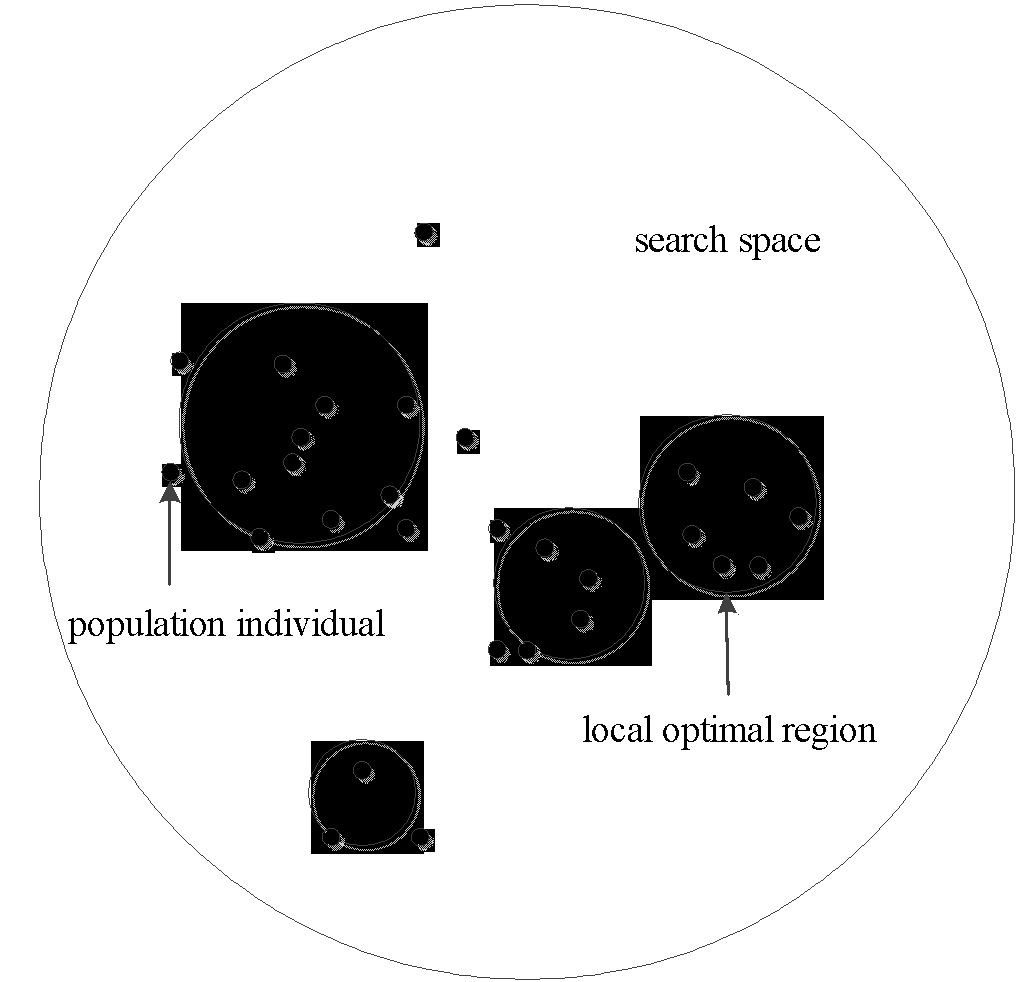Figure 2:  Schematic diagram of the distribution of population individuals obtained by multiple short time searches for GTA-WTA problem using the GA

As shown in Figure 2, the majority of the population individuals are distributed in the local optimal regions and its adjacent regions, and only a few individuals are far from the local optimal regions. So the approximate location of the local optimal regions can be detected by the population individuals. Although the population individuals are in the local optimal regions and its adjacent regions, it is difficult to detect the local optimal regions through a single individual; it can be better detected and represented by a group of densely distributed individuals. In this study, the AP clustering algorithm was used to cluster the population individuals, and the region where the cluster was obtained was used as the local optimal region. In the clustering by the AP clustering algorithm, the element values in the parameter similarity matrix S were assigned as the Euclidean distance between the population individuals.

For the individual xi, xi=(xi1, xi2, …, xiD), i=1, 2, …, n, D is the dimension of the individual, and the Euclidean distance Dij of individual xj is as follows:(3)

According to equation (3), the similarity matrix S can be expressed as follows:(4)

The GA and the AP clustering algorithm can be used to detect the approximate distribution of the local optimal regions in the search space, obtaining a large number of population individuals distributed in each local optimal region, at the same time, obtaining the corresponding local optimal regions to which these individuals belong.

3.2  Initial population optimization

The optimization of the PSO algorithm begins with the initial population. If there are particles relatively close to the optimal solution in the initial population, the optimal solution can be quickly searched. And if the particles are mostly distributed in each local optimal region, the PSO-MDC method can quickly search for the optimums in each local optimal region, which greatly improves the optimization speed. The population initialization method of the PSO algorithm makes it difficult to generate a good initial population. For the deterministic generation of an initial population that is superior and distributed in each local optimal region, the initialization method for the population particles was changed in this study.

After the GA has repeatedly searched the search space for a short time, the population individuals are mostly distributed in each local optimal region and some better individuals; they can be used as the initial population of the PSO algorithm. However, the population size of the PSO algorithm is small, and some of the Euclidean distances between the obtained population individuals are relatively small which do not need to simultaneously exist. Therefore, some densely distributed particles in the initial population can be deleted. The deletion is based on the congestion distance of the particles. The smaller the congestion distance, the more likely the particle is to be deleted. The congestion distance of a particle is the sum of the Euclidean distances of its two closest neighboring particles. The smaller this value is, the denser the particles. The congestion distance Ci of xi is as follows:(5)

In equation (5), xj and xk are the closest particles to particle xi.

For the two closest particles in the population, the particle with smallest congestion distance is deleted. As shown in Figure 3, particles A and B are closest to each other, particles C and D are the adjacent particles of particles A and B, respectively, and the congestion distance of particle B is significantly larger than that of particle A; thus, particle A should be deleted.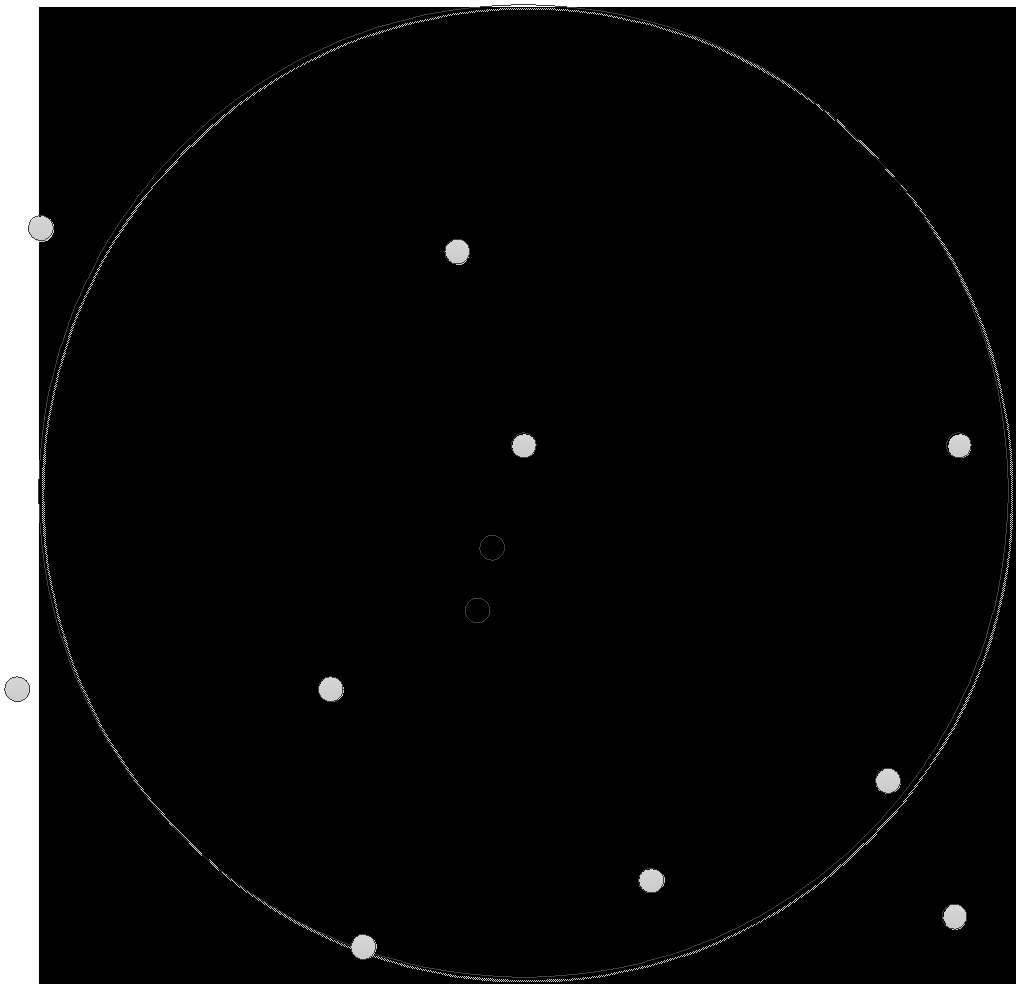Figure 3: Schematic diagram of individual deletion based on congestion distance

The specific procedures for the initial population optimization are as follows:

1. Construct an initial population. Use the GA to repeatedly search the search space of the problem for a short time. All the obtained population individuals are used as the initial population.
2. Sort the population particles. Calculate the Euclidean distance between the particles in the same local optimal region and rearrange all the “particle pairs” in the search space in ascending order of the Euclidean distance between the particles. The “particle pair” refers to the two particles involved in the Euclidean distance calculation.
3. Calculate the congestion distance. Calculate the congestion distance of the particles using equation (5).
4. Delete the intensive particles. Compare the congestion distances of the two particles with the closest European distance and delete the particle with the smaller congestion distance. Repeat this step until the number of particles meets the population size requirement.

3.3 Optimization with multidirectional competition of the particles

The particles of the PSO algorithm update the search position by two “extreme values”. The first is the best solution found by the particle itself, that is, the individual extremum pbest; the other extremum is the best solution currently found in the entire population, that is, the global extremum gbest. After knowing these two extreme values, the search speed and position of particle xi in the d-dimensional direction are updated as follows:(6)(7)

In equations (6) and (7), in the d-dimensional direction of the search space,is the current position of the particle;is the current velocity of the particle;is the position of the particle after being updated;is the velocity of the particle after being updated;is the best solution found by the particle itself;is the best solution currently found for the entire population; w is the inertia weight; c1 and c2 are the acceleration coefficients; r1 and r2 are the random numbers in [0, 1].

The particles are updated based on equations (6) and (7), which can make full use of the optimization information searched by themself and all particles, and increases the probability of the particle reaching a better solution. However, the search tends toward the direction of the optimal solution of the population. When there are multiple local optimal regions in the search space, and there is no global optimal solution in the region where the optimal solution is currently searched, the search can easily fall into a local optimum. If the particles can search in each local optimal region, the search can effectively avoid falling into a local optimum and accelerate the optimization. Therefore, a competition factor is introduced in equation (6), allowing the particles to perform the optimization search in multiple local optimal regions. After introducing the competition factor, equation (6) can be modified as follows:(8)

In equation (8), c3 is the acceleration coefficient,; r3 is a random number in [0, 1]; and lid is the best solution found in the local optimal region where the particle is located, which is called a local extremum.

Equation (8) introduces the particle competition term of, which guides the particle to search toward the global extremum, and also provides a certain probability to search for a better solution in the local optimal region, so that the optimization of particles can be performed in each local optimal region, thus effectively preventing the optimization of particles from falling into a certain local optimal region.

With the update iteration of the population particles, the position changes dynamically. Some particles remain in the local optimal region, while others enter other local optimal regions. To determine the local optimal region of each generation of particles, after each update of the population, the AP clustering algorithm is used to determine the local optimal region of the particles.

3.4  Integer coding based on the attacking target

The GA and PSO algorithm must encode the position of the solution when solving the GTA-WTA problem. The coding method should not only represent the solution of the problem and attempt to satisfy the constraints set in the model but should reduce the length of the code as much as possible. For this purpose, an integer coding method based on the attacking target was designed. The coding structure is shown in Figure 4. In Figure 4, the length of the code is, that is, the dimension is; where, P1, P2, …, and PN×1 represent the allocation of all types of weapons for target 1, PN+1, PN+2, …, and PN×2 represent the allocation of all types of weapons for target 2, and PN×(T-1)+1, PN×(T-2)+1, …, and PN×T represent the allocation of all types of weapons for target T. This coding method does not distinguish the order of attack on the same target for the same type of weapon, and its weapon usage was represented by only one code, which greatly reduced the length of the code.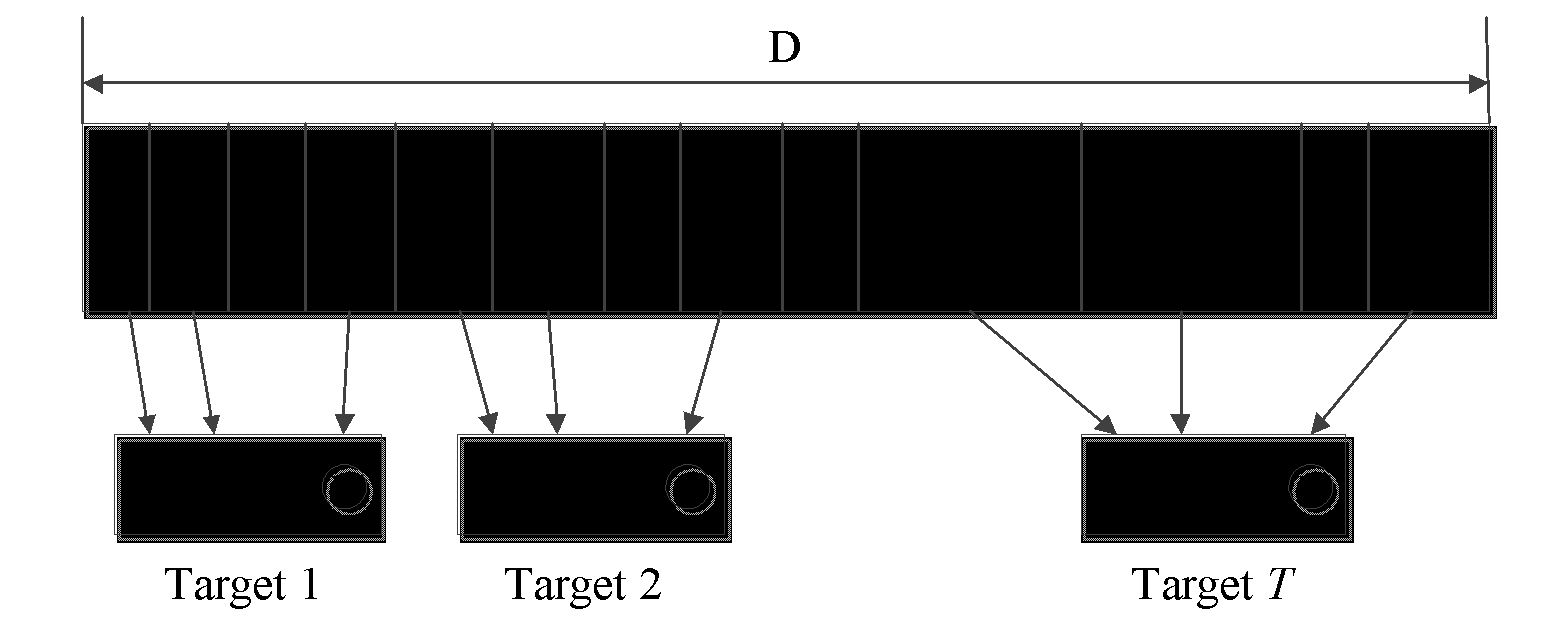Figure 4:  Diagram of the coding structure for the GTA-WTA problem

3.5 Solving procedures of the improved PSO algorithm for the GTA-WTA problem

The design for the PSO-MDC method was illustrated in Sections 3.1 through 3.4. The procedures for solving the GTA-WTA problem can be summarized as follows:

1. Code the problem solution. In the GTA-WTA problem, the individuals of the GA and the particles of the PSO algorithm are encoded by the coding method designed in Section 3.4.
2. Detect the local optimal regions. Use the GA and AP clustering algorithm to detect the local optimal regions of the search space and collect the population individuals distributed in each local optimal region.
3. Optimize the initial population. Use the collected population individuals as the initial population of the PSO algorithm and delete some densely distributed particles based on the congestion distance.
4. Optimize the particles with multidirectional competition. Based on equations (7) and (8), continuously update the velocity and position of the particles until the condition for iterative termination is reached. After each update, use the AP clustering algorithm to determine the local optimal regions where the particles are located.
1. CASE SIMULATION AND ANALYSIS OF THE GTA-WTA PROBLEM

4.1  GTA-WTA problem case

For the typical GTA-WTA problem, we describe four different cases under the same background, based on which we make comparisons.

Case background: A total of five different types of weapons are used, and the value coefficient (unit: million) of each type of weapon and the total investment amount in each case are shown in Table 1. Six types of ground targets are to be attacked, and the number of targets in each case is listed in Table 2. The damage probability of each type of weapon to each type of target is shown in Table 3. It is required that the average damage coefficient on each type of target be 0.8, 0.8, 0.9, 0.85, 0.8 and 0.9. Based on the above conditions, we try to solve the best weapon allocation scheme.

Table 1: The value and total amount of all types of weapons

 Weapon   type Total amount of weapons Value coefficient of weapon Case 1 Case 2 Case 3 Case 4 W1 5 10 15 20 8 W2 5 5 10 15 7 W3 5 10 15 20 6 W4 10 10 20 25 5 W5 5 10 15 20 4

Table 2:  Target type and quantity

 Target type Target quantity Case 1 Case 2 Case 3 Case 4 T1 2 3 4 5 T2 1 3 4 5 T3 1 3 4 5 T4 1 3 4 5 T5 1 2 4 6 T6 2 2 4 6

Table 3: Amage probability of weapon to target

 Target type Weapon type W1 W2 W3 W4 W5 T1 0.85 0.6 0.5 0.45 0.4 T2 0.46 0.35 0.6 0.5 0.35 T3 0.4 0.32 0.6 0.5 0.4 T4 0.8 0.7 0.65 0.5 0.4 T5 0.4 0.3 0.85 0.7 0.6 T6 0.61 0.45 0.75 0.6 0.5

4.2 Simulation and analysis of the GTA-WTA problem

In order to verify that the method proposed in this study can further improve the efficiency of solving GTA-WTA problem, and obtain a better allocation scheme. For the above case, under the same hardware and software condition (win7 x64, Intel (R) Xeon (R) CPU E3-1240 v3 @3.4 GHz, RAM 16 GB), we used MATLAB software to program and simulate the GTA-WTA problem using the improved PSO algorithm of , the improved GA of , and the method proposed in this study, and recorded the optimization time (unit: second) and the weapon consumption value of the three methods.

4.2.1 The optimization time and weapon consumption value of the GTA-WTA problem

Tables 4 and 5 record the results of the five shortest and longest time-consuming simulations, respectively, using the three methods for cases 1 and 4 in 100 simulations.

Table 4: Optimization time and weapon consumption value of the three improved algorithm for case 1

 noun optimization time weapon consumption value the improved PSO algorithm the improved GA the proposed method the improved PSO algorithm the improved GA the proposed method 1 8.2 7.8 7.3 89 88 84 2 8.3 7.8 7.4 85 91 85 3 8.3 7.9 7.5 92 95 83 4 8.4 7.9 7.6 90 89 88 5 8.4 7.9 7.6 93 93 86 6 26.7 25.1 13.8 90 90 85 7 28.6 32.9 14.0 88 91 89 8 32.4 63.5 14.1 87 97 88 9 78.5 90.8 14.6 94 96 84 10 101.3 98.2 14.9 85 102 86

Table 5: Optimization time and weapon consumption value of the three improved algorithm for case 4

 noun optimization time weapon consumption value the improved PSO algorithm the improved GA the proposed method the improved PSO algorithm the improved GA the proposed method 1 158.6 160.4 76.3 462 458 374 2 163.4 163.2 78.9 11111415 471 398 3 163.8 166.5 79.6 454 496 413 4 171.9 170.3 82.5 476 430 426 5 174.3 172.6 84.1 432 441 380 6 1171.5 1051.5 175.3 438 423 375 7 1234.8 1196.4 179.8 491 485 396 8 1267.5 1226.5 180.5 413 478 416 9 1623.6 1432.7 186.7 496 416 403 10 1754.1 1500.2 190.4 474 489 390

According to Tables 4 and 5, it can be seen that in 100 simulation experiments, both the improved PSO algorithm and the improved GA exist the case that the optimization time is too long. In solving case 1, the longest optimization time of the improved PSO algorithm is 12 times the shortest optimization time, and the longest optimization time of the improved GA is 13 times the shortest optimization time. The longest optimization time of the method proposed in this study is only twice as long as the shortest optimization time, and the shortest optimization time of the method proposed in this study is shorter than that of the other two methods. In solving case 4, the longest optimization time of the improved PSO algorithm is 11 times the shortest optimization time, and the longest optimization time of the improved GA is 9 times the shortest optimization time. The longest optimization time of the method proposed in this study is only 2.5 times the shortest optimization time, and the shortest optimization time of the improved PSO algorithm and the improved GA are much longer (2 times) than that of the method proposed in this study. The improved PSO and improved GA algorithms required significant time for optimization because they were trapped in the local optimal regions during the optimization process, requiring considerable time to escape. The method proposed in this study benefits from the effective avoidance of the local optimization for the algorithm in the optimization process, so that the difference between the longest and shortest optimization times is small. By comparing and analyzing the optimization times of the three methods, it is known that the method proposed in this study could effectively solve the occasional lengthy algorithm optimization time problem; the larger the problem, the more obvious the effect.

As shown in Tables 4 and 5, the weapon consumption value optimized by the method in this study is less than those by the improved PSO algorithm and the improved GA. In case 1, the weapon consumption value optimized by the method proposed in this study is slightly less than those of the other two methods; in case 4, the weapon consumption value optimized by the method proposed in this study is much smaller than those of the other two methods. This demonstrated that the method proposed in this study can further improve the quality of the solution.

Based on the above comparative analysis, it can be concluded that the method in this study can effectively solve the occasional lengthy algorithm optimization time problem and further improve the quality of the solution; the larger the GTA-WTA problem, the more obvious the effect.

Tables 4 and 5 only list the optimization times of 10 simulations for the three methods. To more intuitively and comprehensively show the specific situation of the optimization times of the three methods in the 100 simulations, the number of times the optimization times by the different methods occurs in each given time range is statistically analyzed and plotted as an optimization time histogram, as shown in Figures 5 and 6.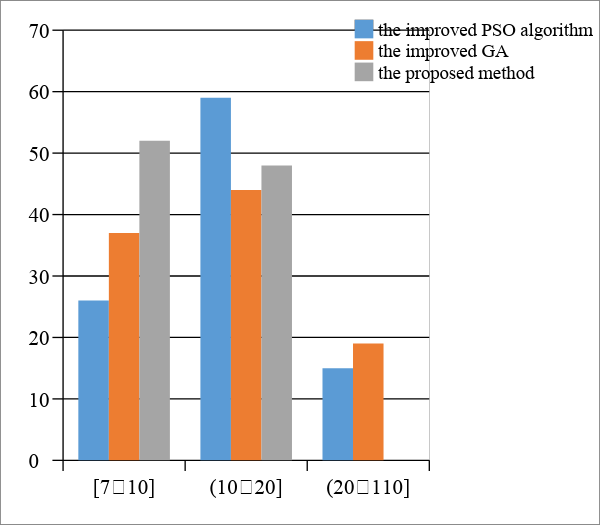Figure 5: The histogram of the optimization times of the three methods for case 1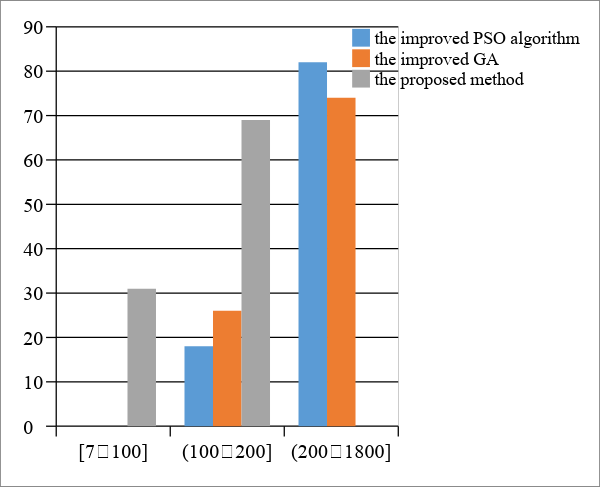Figure 6:  The histogram of the optimization times of the three methods for case 4

As shown in Figure 5, in the 100 simulation experiments of the case 1 problem, the optimization time of the method proposed in this study is between 7 and 20 seconds, primarily less than 10 seconds; the optimization time of the improved PSO algorithm and the improved GA is between 7 and 110 seconds, primarily more than 10 seconds with some instances more than 20 seconds. As shown in Figure 6, in the 100 simulation experiments of the case 4 problem, the optimization time of the method proposed in this study is between 7 and 200 seconds, primarily between 100 and 200 seconds; the optimization time of the improved PSO algorithm and the improved GA is between 7 and 1800 seconds, primarily more than 200 seconds with some instances as great as 1800 seconds. The above comparison showed that the method proposed in this study has a short optimization time and good robustness and can effectively avoid the problem that the optimization time is occasionally too long.

4.2.2 Average optimization times and average weapon consumption values of the GTA-WTA problem

As verified in Section 4.2.1, the method proposed in this study can effectively solve the problem that the optimization time of the algorithm is occasionally too long, and can also improve the quality of the solution. To verify whether the method proposed in this study can effectively shorten the optimization time, and further verify whether it can improve the quality of the solution, the averages of the optimization times and the weapon consumption values of the 100 simulations for cases 1 through 4 by the three methods were compared and analyzed, as shown in Table 6. In Table 6, the “number of variables” is the product of the number of weapon types and the number of targets; the “value of minimal weapon consumption” is the value of the minimal weapon to be consumed to meet the specified destruction and damage requirement; the “average optimization time” is the average of optimization times for 100 simulations; and the “average weapon consumption value” is the average value of the weapon consumption for 100 simulations.

Table 6:  Average Optimization Times and Average Weapon Consumption Values of the Optimizations by the three Methods

 case number of variables value of minimal weapon consumption average optimization time average weapon consumption value the improved PSO algorithm the improved GA the proposed method the improved PSO algorithm the improved GA the proposed method case 1 40 83 12.8 11.6 9.8 91.3 93.7 85.6 case 2 80 173 48.3 44.8 25.4 201.9 206.5 188.3 case 3 120 250 174.1 163.2 70.3 320.4 331.9 286.5 case 4 160 327 424.6 392.7 138.6 448.5 460.2 395.1

As shown in Table 6, compared with the weapon consumption value and optimization time of the improved PSO algorithm and the improved GA, the method proposed in this study has a smaller weapon consumption value and shorter optimization time; this effect become more obvious when the problem is larger. When the number of variables is 40, the average optimization time of the three methods is not much different. When the number of variables is 80 and 120, the average optimization time of the improved PSO algorithm and the improved GA rapidly increase to approximately twice that of the method proposed in this study. When the number of variables is 160, the average optimization time of the improved PSO algorithm and the improved GA rapidly increase to approximately three times that of the method proposed in this study. The comparison showed that the method proposed in this study can effectively shorten the optimization time, further improve the quality of the solution; the larger the problem, the more obvious the effect.

To more intuitively present and analyze the superiority of the method proposed in this study, the average optimization times and the average weapon consumption values in Table 6 are plotted separately, as shown in Figures 7 and 8.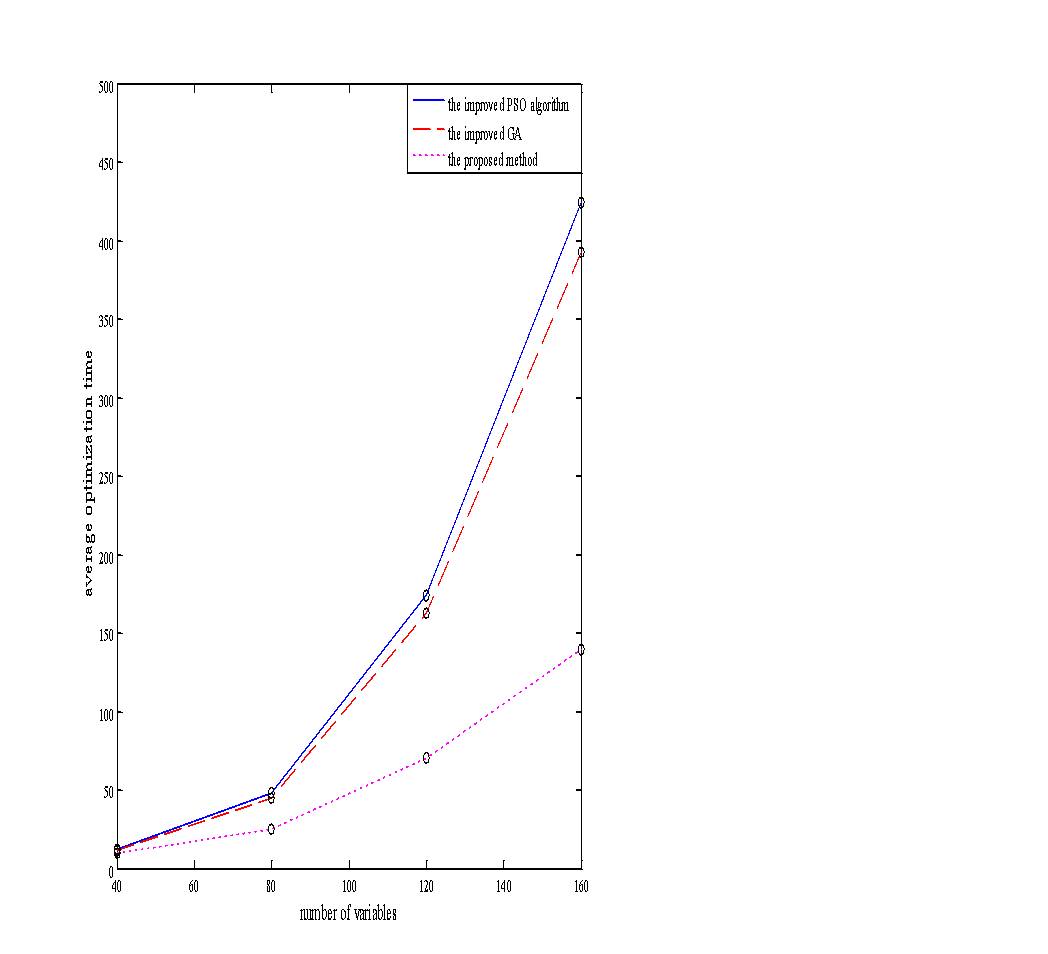Figure 7:  Average Optimization Times of the three Methods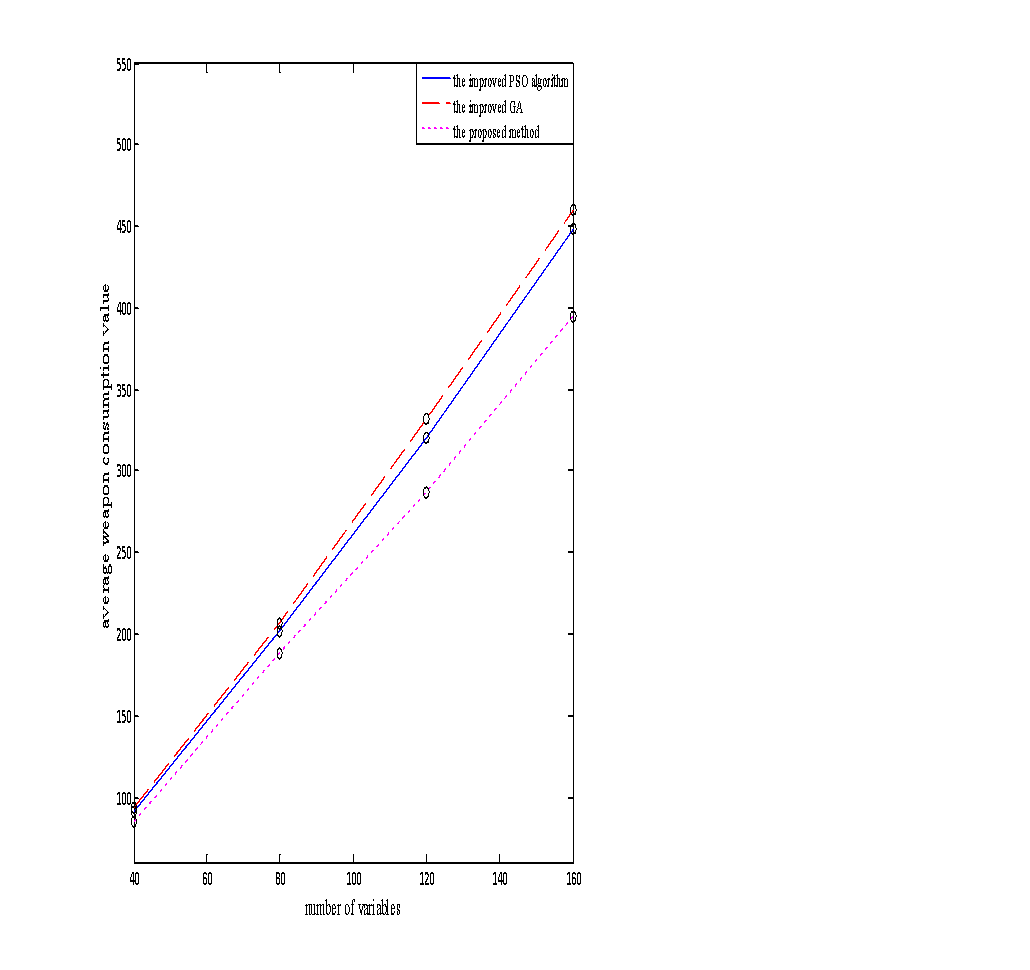Figure 8: Average weapon consumption values of the optimizations by the three methods

As shown in Figure 7, with an increase in the number of variables, the average optimization time and the slope of the curve of the three methods increase. However, the average optimization time and the slope of the curve of the method proposed in this study are always smaller than those of the other two methods. As shown in Figure 8, the average weapon consumption value of the method proposed in this study is always smaller than those of the other two methods. With an increase in the number of variables, the difference in the average weapon consumption value also increase. The comparative analysis showed that the optimization performance of the method proposed in this study was better than those of the other two methods; the larger the problem, the more obvious the effect.

Based on the above comparison and analysis, the method proposed in this study can further improve the computational efficiency and solution quality of GTA-WTA problems; the larger the problem, the more obvious the advantage.

V.    CONCLUSION

To address the problem that the optimization of an intelligent algorithm occasionally requires too much time to solve a GTA-WTA problem, this study proposed a particle swarm optimization method with multidirectional competition. This method uses the GA and the AP clustering algorithm to detect the local optimal regions of the search space, and determines the better solution of each local optimal region to form the initial population of the PSO algorithm. By introducing a multidirectional competition factor in the velocity update of the PSO algorithm, the particles are guided to compete in multiple directions, thus preventing the algorithm from falling into the local optimum and accelerating the optimization. The results of simulation experiments and comparative analyses showed that this method could effectively solve the problem that the optimization of the algorithm occasionally requires too much time, and it has obvious advantages in the computational efficiency and the quality of the solution; the larger the GTA-WTA problem, the more obvious the advantage.

ACKNOWLEDGMENT

This work was jointly supported by the National Natural Science Foundation for Young Scientists of China (Grant No. 61403397, 61202332), and the Natural Science Foundation of Shanxi Province, China (Grant No. 2015JM6313).

REFERENCES

1. Ju Zhang. Research on Weapon Target Assignment Problem [C]. Proceedings of the 3rd China Conference on Command and Control，2015：762-764.
2. Jun Huang. Research on the Model of Weapon Target Assignment [D]. Wuhan: Huazhong University of Science & Technology，2016.
3. Bin Xin，Yipeng Wang，Jie Chen. An Efficient Marginal-Return-Based Constructive Heuristic to Solve the Sensor-Weapon-Target Assignment Problem [J]. IEEE Transactions on Systems, Man, and Cybernetics: Systems，2018：1-12.
4. Xiaoyang Li，Deyun Zhou，Qian Pan，et al. Weapon-Target Assignment Problem by Multiobjective Evolutionary Algorithm Based on Decomposition [J]. Complexity，2018：1-19.
5. Vitaly Shalumov，Tal Shima. Weapon -Target-Allocation Strategies in Multiagent Target-Missile-Defender Engagement [J]. Journal of Guidance, Control, and Dynamic，2017，40（10）：2452-2464.
6. Choi Yong Ho，Lee Young Hoon，Kim Ji Eun. Comparative Study on Performance of Metaheuristics forWeapon-Target Assignment Problem [J]. Journal of the Korea Institute of Military Science and Technology，2017，20（3)：441-453.
7. Krishnamoorthy Kalyanam，David Casbeer，Meir Pachter. Monotone Optimal Threshold Feedback Policy for Sequential Weapon Target Assignment [J]. Journal of Aerospace Information Systems，2017，14（1）：68-72.
8. Yunzhi Zhang，Gang Wang，Xiaoyang Gao，et al. An Improved Cuckoo Search Algorithm for Target Assignment [J]. Proceedings of the 2nd International Conference on Mech- atronics Engineering, and Infoemation, and Information Technology，2017，70：279- 284.
9. Mônica De Rezende，Beatriz S. L. P De Lim，Solange Guimarães. A Greedy Ant Colony System for Defensive Resource Assignment Problems [J]. Applied Artificial Intelligence，2018，38（2）：138-152.
1. Kyle Volle，Jonathan Rogers，Kevin Brink. Decentralized Cooperative Control Methods for the Modified Weapon-Target Assignment Problem [J]. Journal of Guidance, Control, and Dynamics，2016，39（9）：1934-1948.
2. Yizhe Chang，Zhanwu Li，Yingxin Kou， et al. A New Approach to Weapon-Target Assignment in Cooperative Air Combat [J]. Mathematical Problems in Engineering，2017：1-17.
3. Juan Li，Jie Chen，Bin Xin， et al. Efficient Multi-objective Evolutionary Algorithms for Solving the Multi-stage Weapon Target Assignment Problem: A Comparison Study [J]. 2017 IEEE Congress on Evolutionary Computation，2017：435-442.
4. Doo-Hyun Cho，Han-Lim Choi. Greedy Maximization for Asset-Based Weapon-Target Assignment with Time-Dependent Rewards [J]. Cooperative Control of Multi-Agent Systems: Theory and Applications，2017：115-138.
5. Zhichao Liu，Zhangsong Shi，Ling Wu，et al. Solving Cooperative Anti-Missile Weapon-Target Assignment Problems using Hybrid Algorithms based on Particle Swarm and Tabu Search [J]. International Conference on Computer Science and Application Engineering，2017：898-906.
6. Deyun Zhou，Xiaoyang Li，Qian Pan，et al. Multiobjective Weapon-Target Assignment Problem by Two-Stage Evolutionary Multiobjective Particle Swarm Optimization [C]. 2016 IEEE International Conference on Information and Automation (ICIA)，2016：3132-3139.
7. Shunhong Wang，Qisong Yang，Ranhui Wang，et al. Particle Swarm Optimization Based Weapon-target Assignment for Attacking Ground Targets [J]. Electronics Optics ＆ Control，2017，24（3）：36-40.
8. Jun Wang，Pengcheng Luo，Jinglun Zhou. A Memetic Algorithm for Constrainted Weapon Target Assignment Problems [C]. 2017 2nd IEEE International Conference on Computation Intelligence and Applications (ICCIA)，2017：182-188.
9. Shuangshuang Liu，Ruiming Xu，Junjie Pan. Research on the Modeling and Solving of the Joint Long-range Strike Weapon Target Assignment Problem Based on the Niche Bat Algorithm [J]. Journal of Equipment Acade，2017，28（2）：93-98.
10. J Kennedy，R Eberhart． Particle Swarm Optimization [C]. 1995 IEEE International Conference on Neural Networks，1995: 1942-1948.
11. SP Lloyd，HS Witsenhausen. Weapon Allocations is NP-complete [C]. IEEE Summer Conference on Simulation，1986：88-95．
12. Yanzhao Zhou. Improvement and Application of Adaptive Genetic Algorithm [D]. Fuxin City：Liaoning Project Technology University，2013．#### For Authors

/symposiums/webinars, networking opportunities, and privileged benefits.
Authors may submit research manuscript or paper without being an existing member of LJP. Once a non-member author submits a research paper he/she becomes a part of "Provisional Author Membership".

Know more#### For Institutions

Society flourish when two institutions come together." Organizations, research institutes, and universities can join LJP Subscription membership or privileged "Fellow Membership" membership facilitating researchers to publish their work with us, become peer reviewers and join us on Advisory Board.

Know more#### For Subscribers

Subscribe to distinguished STM (scientific, technical, and medical) publisher. Subscription membership is available for individuals universities and institutions (print & online). Subscribers can access journals from our libraries, published in different formats like Printed Hardcopy, Interactive PDFs, EPUBs, eBooks, indexable documents and the author managed dynamic live web page articles, LaTeX, PDFs etc.

Know more